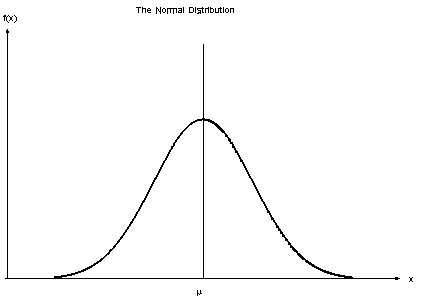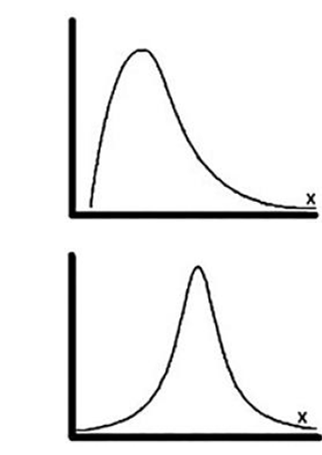# Assessing Normality of Data using SPSS AMOS

## What is Normality

A probability distribution that is symmetric about the mean, showing that data near the mean are more frequent in occurrence than data far from the mean.

Normality is assessed using

• Skewness
• Kurtosis## Skewness and Kurtosis

• Skew—is the tilt in the distribution. Maybe on the left or on the right (Top graph in the figure).
• Kurtosis—is the peakedness of a distribution. This heaviness or lightness in the tails usually means that your data looks flatter (or less flat) compared to the normal distribution (Bottom part of the figure).## Normality Assessment

• The normality assessment is made by assessing the measure of skewness for every item. The absolute value of skewness 1.0 or lower indicates the data is normally distributed.
• However, SEM using the Maximum Likelihood Estimator (MLE) like AMOS is fairly robust to skewness greater than 1.0 in absolute value if the sample size is large and the Critical Region (CR) for the skewness does not exceed 8.0.
• Meaning, the researcher could proceed into further analysis (SEM) since the estimator used is MLE. Normally the sample size greater than 200 is considered large enough in MLE even though the data distribution is slightly non-normal.
• Thus, for sample size greater than 200, the researcher could proceed further analysis with the absolute skewness up to +/-2 although, some experts have suggested a value of +/-3.
• Another method for normality assessment is by looking at the kurtosis statistic. However, SEM using Maximum Likelihood Estimator (MLE) is also robust to kurtosis violations of multivariate normality as long the sample size is large.
• For kurtosis, the range is −10 to +10 to still be considered normally distributed (Collier, 2020). Based on our results, we can see that both the skew and kurtosis are in an acceptable range to be considered “normal”.

## Normality Assessment - Mahalanobis Distance

• If the distribution is found to depart from normality, the researcher could assess the Mahalanobis distance to identify for the potential outliers in dataset.
• AMOS computes the distance for every observation in dataset from the centroid. The centroid is the center of all data distribution.
• It tabulates the distance of potential outliers from the centroid together with the probability for an observation suspected to be an outlier in the first column and the probability that an observation of similar extremity would occur given a multivariate normal population (the second column).
• The outlier occurs when the distance of certain observation is too far compared to the majority other observations in a dataset.
• The deletion of few extreme outliers in the model might improve the multivariate normality. Once the outlier is identified, the researcher could go back to dataset and get them deleted (based on the observation number).
• The new measurement model is re-specified using the cleaned dataset. The process could be repeated. However, there is no necessity to examine Mahanolobis Distance if the non-normality issue does not arise.
• This statistic represents the squared distance from the centroid of a data set. The bigger the distance, the farther the item is from the mean distribution.
• AMOS also presents two additional statistics, p1 and p2.
• A good rule of thumb is that If you have p1 and p2 values that are less than .001, these are cases denoted as outliers (Collier, 2020).

## Failure to Satisfy Normality Assumption

• As a summary, in the case when the normality assumption is not fulfilled, the researchers still have many options to take.
• One of them is to remove the non-normal items from the measurement model (based on the measure of skewness) and continue with the analysis.
• Another option is to remove the farthest observation from the center (outlier) of distribution.
• However, the most popular method lately is to continue with the analysis with MLE (without deleting any item and also without removing any observation) and re-confirm the result of analysis through Bootstrapping.
• Bootstrapping is the re-sampling process on the existing dataset using the method of sampling with replacement. The statistical procedure would compute the mean and standard deviation for every sample of size n to create the new sampling distribution.
• The researcher could instruct Amos to collect 1000 random sample from the dataset and re-do the analysis.
• Since the sample size is large (1000), the new sampling distribution would be closer to normal distribution. Amos would analyze the Bootstrapping data and produce the confidence intervals as well as the significance for every parameter involved in the analysis.
• The researcher could compare the actual results with the bootstrapped results to confirm the analysis. If the results differ, the bootstrapped result will be acceptable.

## Reference

Collier, J. E. (2020). Applied structural equation modeling using AMOS: Basic to advanced techniques. Routledge.